December 17, 2021

# Class 10 Maths Chapter 13 Surface Areas And Volumes

We encountered surface areas and volumes in chapter 13 in class 9th. We learned formulas for surface area and volume for different shapes like cubes, cuboids, cones, cylinders, spheres, and hemispheres. There we practiced and solved questions based on these shapes only, but in class 10  maths chapter 13 surface areas and volumes, we will learn how to calculate the surface area and volume of the combination of all these shapes.

Along with the combination of shapes, we will learn the conversion of solids from one shape to another and the frustum of a cone is a new topic that you will learn in this chapter.

## Class 10 Maths Chapter 13 Surface Areas And Volumes

A lot of formulas will be used in this chapter, therefore, getting familiar with them is inevitable. But, first, we must know the meaning of all the terms that will be used throughout chapter 13. So, let’s begin with the terminologies of this chapter.

Plane & solid figures

The figures that can be drawn on the plane surfaces like notebooks are called plane figures. e.g circle, square, rectangle, etc. Now, imagine that we have lots of squares of the same size, and we stack them over each other, then we will get a solid figure called a cube. So, to put it in simple words, solid figures are just the 3d version of plane figures. e.g cube, cuboid, cone, etc.

Surface area and its type

In class 10 maths chapter 13 surface areas and volumes, the surface area as the name suggests is the area covered by the surface of a shape or figure. There are two types of surface area i.e total surface area and lateral or curved surface area.

The total area occupied by all the surfaces of a shape or figure is called its total surface area. e.g a cube has 6 faces, so the sum of the area of all the faces will be its total surface area.

The lateral surface area is also referred to as curved surface area in a general sense, but, they are not actually the same. Lateral surface area means the surface area of faces that are on sides (excluding top and bottom faces). e.g the lateral surface of a cube will be the sum of only 4 sides because we will leave the faces that are on top and bottom.

Whereas the curved surface area is used for shapes that have a curve in them e.g cylinder. Just like lateral surface area, in the curved surface area, we do not calculate the area of the top and bottom of shapes. e.g if we are to calculate the CSA of a cylinder, then we will exclude the top and bottom.

In Lehman terms, LSA is used in simple solid figures, whereas, CSA is used for solid figures with curves.

What is Volume in class 10 maths chapter 13 surface areas and volumes

The volume is the capacity of a solid figure. The measurement of capacity for different solid figures can be different.

e.g for a cube, the measure of capacity is the number of small cubes that can be held inside it, whereas, for a cylindrical tank, the measure of capacity will be the amount of water or other liquid it can hold. Volume is sometimes also referred to as the capacity of the container.

### Class 10 Maths Chapter 13 Surface Areas And Volumes

It may look unnecessary to explain common terms like surface areas and volumes to students of class 10th, but it is always a good practice to first refresh the basics of the topic before going to its advanced part. 1 year is a big gap, and forgetting things is a very normal thing to happen. So, here is the list of all the formulas that we have learned in class 9th and will continue to use in class 10 maths chapter 13 surface areas and volumes.

CBSE class 10 maths chapter 13 surface areas and volumes – formulas for all shapes
Solid Shapes TSA LSA/CSA Volume
Cuboid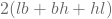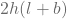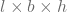Cube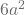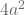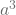Cone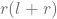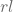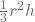Cylinder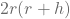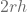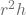Sphere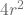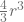Hemisphere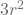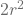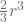l → length or slant height, b → breadth, h → height, a → sides, r → radius

All these formulas will be used when you will get to the problem-solving part of this chapter.

#### Class 10 Maths Chapter 13 Surface Areas And Volumes

Now that we are through the basic terminology and formulas that are needed to practice this chapter effectively, we can now move on to actually practice this chapter.

# Surface area of a combination of solids

In the previous class, we practiced problems of surface area related to different shapes, but in all those problems we had to find the surface area of single shapes. But, in class 10 maths chapter 13 surface areas and volumes, we will learn how to calculate the surface area of a combination of shapes i.e not just one shape but combinations of different shapes.

# Volume of a combination of solids

Just like surface areas, in this class, we will learn how to find out the volume of shapes that are actually the combination of different shapes. This chapter is more close to practical life as most of the objects around us are combinations of different shapes instead of being a single shape.

# Conversion of solid from one shape to another

Objects that exist in one shape can be transformed into some other shape, and this section of class 10 maths chapter 13 surface areas and volumes teaches us how we can easily calculate the area, sides, height, etc. of a shape if it is converted into some other shape. e.g how big a sphere that is made after melting a cube will be.

# Frustum of a cone

If we cut a right circular cone horizontally i.e in such a way that the top and bottom of the cone are now separate, then the bottom part of that cone will be called its frustum. So, the frustum of a cone is simply the bottom part of the cone when cut horizontally.

The frustum will always have two circles, one at the top and one at the bottom, and the radii of both the circles will be different. Here are the formulas for surface areas and volume for a frustum.

 slant height of frustum (l)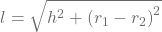Curved surface area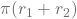Total surface area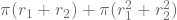Volume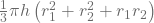So these are all the topics from NCERT book. Make sure to practice class 10 maths chapter 13 surface areas and volumes the best you can because they are going to come in your future classes. So, it’s better to be prepared.